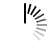Get the size of an ArrayList in Java

The size of an ArrayList can be obtained by using the java.util.ArrayList.size() method as it returns the number of elements in the ArrayList i.e. the size.

A program that demonstrates this is given as follows −

Example

Live Demo

```import java.util.ArrayList;
import java.util.List;
public class Demo {
public static void main(String[] args) {
List aList = new ArrayList();
System.out.println("The size of the ArrayList is: " + aList.size());
}
}```

Output

`The size of the ArrayList is: 5`

Now let us understand the above program.

The ArrayList aList is created. Then ArrayList.add() is used to add the elements to the ArrayList. ArrayList.size() returns the size of the ArrayList and that is displayed. A code snippet which demonstrates this is as follows −

```List aList = new ArrayList();